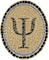Psellos
Contemporary Development With Functional Programming

# OCaml, Objective C, Rule 4

December 4, 2014

I recently spent some time tracking down another problem in an OCaml iOS app. The symptom was that the app would work fine for 5 minutes and 50 seconds, then would crash. The app, named Portland, is very simple; its only input is a periodic categorization of the spatial orientation of the phone. The timing of the crash was quite consistent.

It turns out that the same problem can be demonstrated in OS X. At the risk of revealing just how many errors I make in coding, I thought I’d write up this example also. I can imagine that somebody else might see the problem some day.

Even an OCaml iOS app will have some parts written in Objective C. The error showed up because I wanted to have a table in Objective C holding some OCaml values. I made a tiny example that shows the problem in OS X. Here is the table in Objective C (`table.m`):

``````#include <Foundation/Foundation.h>

#include "caml/memory.h"
#include "caml/alloc.h"

static NSMutableDictionary *g_dict = nil;

static NSString *NSString_val(value sval)
{
return [NSString stringWithCString: String_val(sval)
encoding: NSUTF8StringEncoding];
}

value table_add(value k, value v)
{
CAMLparam2(k, v);

if (g_dict == nil)
g_dict = [NSMutableDictionary dictionary];

NSNumber *val = [NSNumber numberWithLong: v];
[g_dict setObject: val forKey: NSString_val(k)];
CAMLreturn(Val_unit);
}

value table_lookup(value k)
{
CAMLparam1(k);
CAMLlocal1(some);

NSNumber *val;
if ((val = [g_dict objectForKey: NSString_val(k)]) != nil) {
some = caml_alloc_tuple(1);
Store_field(some, 0, [val longValue]);
CAMLreturn(some);
}
CAMLreturn(Val_int(0)); /* None */
}``````

The table associates a string with an OCaml value. You have to imagine that I have some reason to retrieve the OCaml value for a string in the Objective C code. But for this example I’ll look up values from OCaml using `table_lookup()`.

The OCaml main program looks like this (`r4.ml`):

``````external table_add : string -> int list -> unit = "table_add"
external table_lookup : string -> int list option = "table_lookup"

let rec replicate n x = if n <= 0 then [] else x :: replicate (n - 1) x

let rec check iter =
(* Keep checking whether the "four" entry looks right. If not,
* return the iteration number where it fails.
*)
if iter mod 1000000 = 0 then
Printf.printf "iteration %d\n%!" iter;
match table_lookup "four" with
| Some [_; _; _; _] -> check (iter + 1)
| _ -> iter

let main () =
table_add "three" (replicate 3 1);
table_add "four" (replicate 4 1);
let failed_iter = check 1 in
Printf.printf "failed at iteration %d\n" failed_iter

let () = main ()``````

The program creates two entries in the table. The value for `"four"` is the list `[1; 1; 1; 1]`. Then—and you know this means something is very wrong—it fetches the value for `"four"` repeatedly and checks that it has the correct length.

If you compile this and run it on OS X a couple of times, you see the following:

``````\$ uname -rs
Darwin 13.3.0
\$ cc -I /usr/local/lib/ocaml -c table.m
\$ ocamlopt -o r4 -cclib '-framework Foundation' r4.ml table.o
\$ r4
failed at iteration 131067
\$ r4
failed at iteration 131067``````

So, at iteration 131067 the length of the list for `"four"` changes to something other than 4. The first 131066 iterations correspond to the 5 minutes 50 seconds when my iOS app worked fine. Then things go wrong. Note that 131067 is suspiciously close to a power of 2.

You, reader, are possibly way ahead of me and already see what’s wrong. But what I did was work through the problem carefully with lldb. Eventually I figured out that I had broken Rule 4:

Rule 4 Global variables containing values must be registered with the garbage collector using the `caml_register_global_root` function.

In retrospect this is obvious. OCaml values are subject to change at every allocation. But they can’t change if the GC can’t find them, so they need to be registered. The values in the table aren’t registered, so they become invalid at the first GC. You can find Rule 4 and the Other Rules here:

Living in harmony with the garbage collector

One reason it was difficult to code this correctly is that the `NSNumber` wrapper class doesn’t have an interface for getting a pointer to the wrapped-up number. I thought about this for a while and ended up doing the following (corrected `table.m`):

``````#include <Foundation/Foundation.h>

#include "caml/memory.h"
#include "caml/alloc.h"

static NSMutableDictionary *g_dict = nil;

static NSString *NSString_val(value sval)
{
return [NSString stringWithCString: String_val(sval)
encoding: NSUTF8StringEncoding];
}

value table_add(value k, value v)
{
CAMLparam2(k, v);

if (g_dict == nil)
g_dict = [NSMutableDictionary dictionary];

value *vp = malloc(sizeof *vp);
if (vp == NULL)
CAMLreturn(Val_unit); /* No memory for adding to table */
*vp = v;
caml_register_global_root(vp);
NSValue *val = [NSValue valueWithPointer: vp];
[g_dict setObject: val forKey: NSString_val(k)];
CAMLreturn(Val_unit);
}

value table_lookup(value k)
{
CAMLparam1(k);
CAMLlocal1(some);

NSValue *val;
if ((val = [g_dict objectForKey: NSString_val(k)]) != nil) {
some = caml_alloc_tuple(1);
Store_field(some, 0, * (value *) [val pointerValue]);
CAMLreturn(some);
}
CAMLreturn(Val_int(0)); /* None */
}``````

Since I can’t get pointers to wrapped up values, I make pointers myself and wrap them.

If you compile and run this corrected version, it looks like this:

``````\$ cc -I /usr/local/lib/ocaml -c table.m
\$ ocamlopt -o r4 -cclib '-framework Foundation' r4.ml table.o
\$ r4 | head -12
iteration 1000000
iteration 2000000
iteration 3000000
iteration 4000000
iteration 5000000
iteration 6000000
iteration 7000000
iteration 8000000
iteration 9000000
iteration 10000000
iteration 11000000
iteration 12000000``````

I have every reason to believe the corrected iOS app will run until the cows come home 12,000,000 times.

I hope this may help some other lonely OCaml developer who sees a crash after 5 minutes 50 seconds. May we all live in harmony. If you have any comments or sympathy, leave them below or email me at jeffsco@psellos.com.

Posted by: Jeffrey